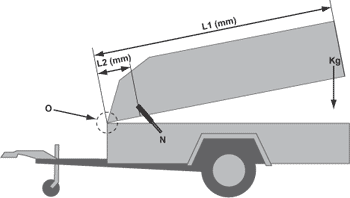# How do I calculate the Newton value for a cover?L1*= Kg (Total weight in kg)*= L2*= N (Number of gas springs) =